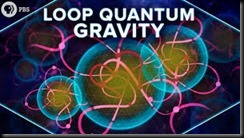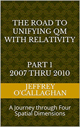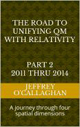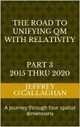There are two ways science attempts to understand or define the behavior of our world. The first is Quantum mechanics or the branch of physics that uses probabilities to define the wave particle duality of existence.  The other is the deterministic universe of Einstein where the interactions of space with time determines the casualty of events in the macroscopic universe we live in.Specifically, the General Theory of Relativity tells us that gravity is a result of a curvature in space-time whose magnitude is directly related to the amount of matter or number of particles contained in given volume of space-time.

While quantum mechanics use probabilistic properties of the wave particle duality of existence to define the position of particles such as protons and elections in a given volume of space.

Since we all live in the same world you would expect the probabilistic approach of quantum mechanics to be compatible with the deterministic one of Einstein.  Unfortunately, they define two different worlds that on the surface appear to be incompatible.  One defines existence in terms of the probabilities associated with the wave particle duality of existence mentioned earlier while the other defines it in terms the deterministic of properties the continuous field of space and time.

Therefore, one could argue the Physicist of Quantum Gravity is the science of explaining the how the probabilities associated the wave particle duality of a quantum existence controls the deterministic universe of gravity as defined by Einstein or show how that universe is responsible for those probabilities in terms of the interaction of space and time.

For example, one can derive the probability of getting a six on a role of dice based on the fact that dice physically has six sides with only one having a six on it.  This is true even though one cannot predict when a six will occur.  In other words. one can show that probability getting a six on the role of a dice is determined by the physical properties of the dice and not that the probably of six occurring is the reason one rolls a six.

Similarly, if one can show the position of a particle is not caused by the probability of finding it there but is caused by a property of space-time one could understand how to connect gravity with the probabilistic world of quantum mechanics.  This is because, as mentioned earlier Einstein defined gravity in terms of the number of particles in given volume of space-time.

For example, in the article “Why is energy/mass quantized?” Oct. 4, 2007 it was shown the wave particle duality of existence define by quantum mechanics can be understood by extrapolating the laws of classical resonance in a three-dimensional environment to an energy wave on a "surface" of a three-dimensional space manifold with respect to a time dimension.

Briefly it showed the four conditions required for resonance to occur in a classical environment, an object, or substance with a natural frequency, a forcing function at the same frequency as its natural frequency, the lack of a damping frequency and the ability for the substance to oscillate spatial would occur in an environment consisting of four-dimensional space-time.

The existence of four-dimensional space-time would give an energy wave the ability to oscillate spatially on a "surface" the third spatial dimension with respect to the time dimension thereby fulfilling one of the requirements for classical resonance to occur.

These oscillations would be caused by an event such as the decay of a subatomic particle or the shifting of an electron in an atomic orbital.  This would force the "surface" of a three-dimensional space manifold to oscillate with the frequency associated with the energy of that event.

The oscillations caused by such an event would serve as forcing function allowing a resonant system or "structure" to be established space.

Therefore, these oscillations in a "surface" of a three-dimensional space manifold would meet the requirements mentioned above for the formation of a resonant system or "structure" in four-dimensional space-time if one extrapolated them to that environment.

Classical mechanics tells us the energy of a resonant system can only take on the discrete or quantized values associated with its fundamental or a harmonic of its fundamental frequency.

Hence, these resonant systems in four-dimensional space-time would be responsible for particle property of existence in the space-time environment of Einstein.

Yet one can also define the boundary conditions responsible for a creating the resonant system or "structure" that earlier defined a particle.

For example, in our three-dimensional world, a point on the two-dimensional surface of paper is confined to that surface.  However, that surface can oscillate up or down with respect to three-dimensional space.

However, the edge of the paper provides a boundary that reflects those oscillation back on itself, thereby creating a resonant wave on the surface of the paper.

Similarly, an energy wave of three-dimensional space would be confined to it however, it could, similar to the surface of the paper oscillate “up” or “down” while moving through time.

However, when it is prevented from moving thought time either by being observed or encountering an object or particle that wave energy will be reflected back on itself, thereby creating a resonant wave on the "surface" three-dimensional space,

In other words, if the wave component of quantum existence is prevented from moving unhindered through time either by an observation or by an interaction with a particle or object it will create a resonant system or structure that defined the quantum properties of existence in the article "Why is energy/mass quantized?".

This shows how, one can explain the wave particle duality quantum existence in terms of an interaction of space with time.

The final step in integrating quantum mechanics with Einstein gravitational theories is to physical connect its probabilistic interoperation of a particles position with the physical properties of space-time as defined by him.

The physics of wave mechanics tells us, due to the continuous properties the energy wave of a quantum system  means it would be distributed throughout "surface" a three-dimensional space manifold with respect to time.

For example, the energy of a vibrating or oscillating ball on a rubber diaphragm would be disturbed over its surface while the magnitude of those vibrations would decrease as one move away from the focal point of the oscillations.

Similarly, if the assumption that wave properties of a quantum existence represents vibrations or oscillations in a "surface" of three-dimensional space, is correct these oscillations would be distributed over the "surface" three-dimensional space while the magnitude of those vibrations would be greatest at the focal point of the oscillations and decreases as one moves away from it.

However as the article, mentioned earlier  “Why is energy/mass quantized?” showed the particle property of existence is a result of a resonant structure formed on the "surface" of a three-dimensional space manifold by its interaction with the time dimension.

Yet the science of Wave Mechanics tells us resonance would most probably occur on the surface of the rubber sheet were the magnitude of the vibrations is greatest and would diminish as one move away from that point.

Similarly, the resonant structure that article associated with a particle properties of existence would most probably be found were the magnitude of the vibrations in a "surface" of a three-dimensional space manifold is greatest and would diminish as one move away from that point.

This explains why in terms of the physical properties of four-dimensional space-time why one must use the probabilities associate with quantum mechanics to determine the exact the position a single particle in space.  However, it allows one integrate the probabilities associated with the quantum mechanical definition of existence with the physical properties gravity because as was mentioned earlier gravity can be defined in terms of the quantity of particles in a given volume of space-time.

In other words, the gravitational force in a given region of space-time will be greater where the probability density of particles as define by quantum mechanics is the greatest.

As was mentioned earlier one can show that probability getting a six on the role of a dice is determined by the physical properties of the dice and not that the probably of six occurring is the reason one rolls a six.

In other word, the Physicists of Quantum Gravity may not be related to the quantum probability of finding a particle in a given region of space-time but to determining reasons why those probabilities are what they are.

Later Jeff

 The Road to Unification part 1 2007 thru 2010Ebook \$8.00 The Road to Unification part 2 2011 thru 2014Ebook \$8.00 The Road to Unification part 3 2015 thru 2020Ebook \$8.00

Name*

Unifying Quantum and Relativistic Theories is based on WordPress platform.